SBVS297B November   2019  – May 2020

PRODUCTION DATA.

1. Features
2. Applications
3. Description
1.     Device Images
4. Revision History
5. Pin Configuration and Functions
6. Specifications
7. Detailed Description
1. 7.1 Overview
2. 7.2 Functional Block Diagram
3. 7.3 Feature Description
1. 7.3.1 Voltage Regulation Features
2. 7.3.2 System Start-Up Features
3. 7.3.3 Internal Protection Features
4. 7.4 Device Functional Modes
8. Application and Implementation
1. 8.1 Application Information
2. 8.2 Typical Application
9. Power Supply Recommendations
10. 10Layout
1. 10.1 Layout Guidelines
2. 10.2 Layout Example
11. 11Device and Documentation Support
1. 11.1 Device Support
1. 11.1.1 Development Support
2. 11.1.2 Device Nomenclature
2. 11.2 Documentation Support
4. 11.4 Support Resources
6. 11.6 Electrostatic Discharge Caution
7. 11.7 Glossary
12. 12Mechanical, Packaging, and Orderable Information

• RPS|12

6.6 Typical Characteristics

at TA = 25°C, VIN = 1.4 V or VIN = VOUT(NOM) + 0.4 V (whichever is greater), VBIAS = open, VOUT(NOM) = 0.8 V, VEN = 1.1 V, COUT = 47 µF, CNR/SS = 0 nF, CFF = 0 nF, and PG pin pulled up to VIN with 100 kΩ (unless otherwise noted)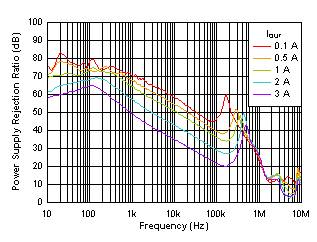VIN = 1.2 V, VBIAS = 5 V, COUT = 47 µF || 10 µF || 10 µF, CNR/SS = 10 nF, CFF = 10 nF
Figure 1. PSRR vs Frequency and IOUT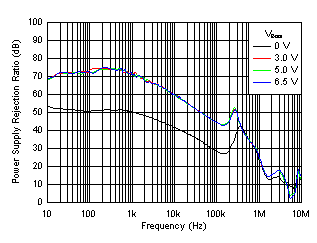VIN = 1.4 V, IOUT = 1 A, COUT = 47 µF || 10 µF || 10 µF, CNR/SS = 10 nF, CFF = 10 nF
Figure 3. PSRR vs Frequency and VBIAS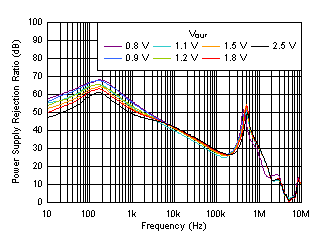VIN = VOUT + 0.4 V, VBIAS = 5.0 V, IOUT = 3 A, COUT = 47 µF || 10 µF || 10 µF, CNR/SS = 10 nF, CFF = 10 nF
Figure 5. PSRR vs Frequency and VOUT With Bias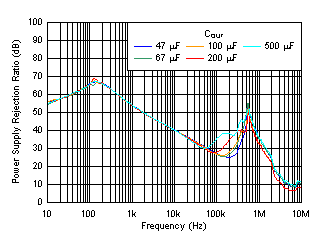VIN = VOUT + 0.4 V, VOUT = 1 V, IOUT = 3 A, CNR/SS = 10 nF, CFF = 10 nF
Figure 7. PSRR vs Frequency and COUT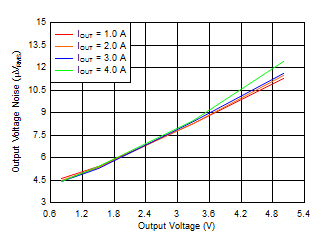VIN = VOUT + 0.4 V and VBIAS = 5 V for VOUT ≤ 2.2 V, COUT = 47 µF || 10 µF || 10 µF, CNR/SS = 10 nF, CFF = 10 nF, RMS noise BW = 10 Hz to 100 kHz
Figure 9. Output Voltage Noise vs Output Voltage and IOUTIOUT = 1 A, COUT = 47 µF || 10 µF || 10 µF, CNR/SS = 10 nF, CFF = 10 nF, RMS noise BW = 10 Hz to 100 kHz
Figure 11. Output Voltage Noise vs Frequency and VIN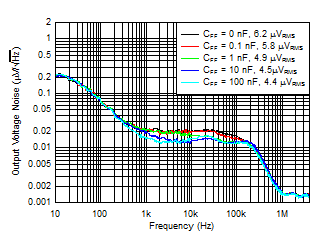VIN = VOUT + 0.4 V, VBIAS = 5 V, IOUT = 4 A, sequencing with a DC/DC converter and PG, COUT = 47 µF || 10 µF || 10 µF, CNR/SS = 10 nF, RMS noise BW = 10 Hz to 100 kHz
Figure 13. Output Voltage Noise vs Frequency and CFF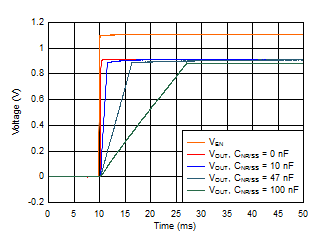VIN = 1.2 V, VOUT = 0.9 V, VBIAS = 5.0 V, IOUT = 4 A, COUT = 47 µF || 10 µF || 10 µF, CFF = 10 nF
Figure 15. Start-Up Waveform vs Time and CNR/SS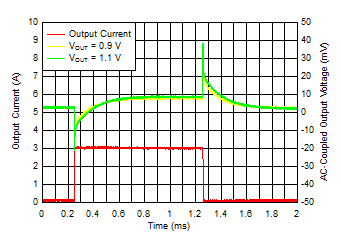IOUT, DC = 100 mA, COUT = 47 µF || 10 µF || 10 µF, CNR/SS = CFF = 10 nF, slew rate = 1 A/µs
Figure 17. Load Transient vs Time and VOUT Without Bias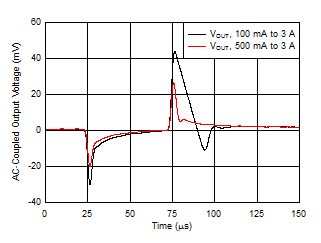VIN = 1.2 V, VBIAS = 5.0 V, COUT = 47 µF || 10 µF || 10 µF, CNR/SS = CFF = 10 nF, slew rate = 1 A/µs
(VOUT = 0.9 V)IOUT = 3 A, VBIAS = 5 V
Figure 21. Dropout Voltage vs Input Voltage With BiasVIN = 1.1 V, VBIAS = 3 V
Figure 23. Dropout Voltage vs Output Current With Bias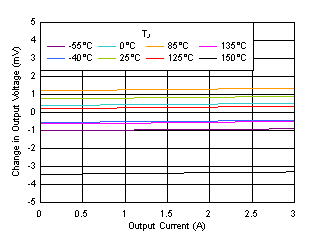VIN = 1.1 V, VBIAS = 5 V
Figure 25. Load Regulation With Bias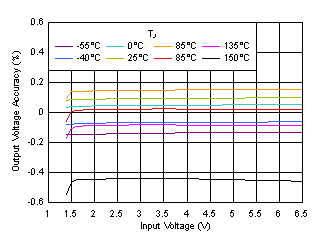VOUT = 0.8 V, VBIAS = 0 V, IOUT = 5 mA
Figure 27. Line Regulation Without BiasVIN = 1.1 V, IOUT = 5 mA
Figure 29. Quiescent Current vs Bias Voltage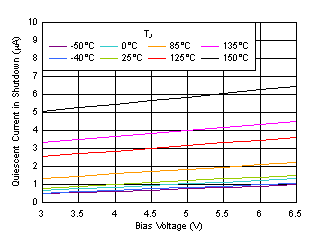VIN = 1.1 V
Figure 31. Shutdown Current vs Bias Voltage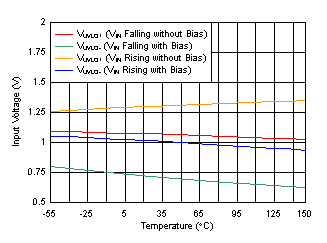Figure 33. VIN UVLO vs Temperature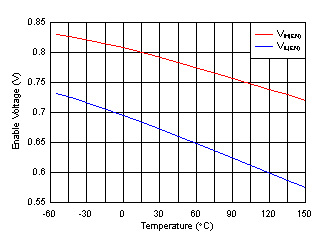VIN = 1.4 V, 6.5 V
Figure 35. Enable Threshold vs TemperatureVIN = 6.5 V
Figure 37. PG Voltage vs PG Current Sink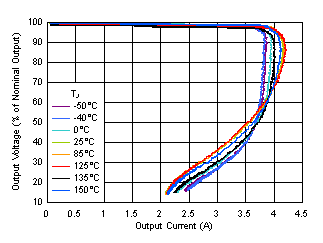VIN = 1.1 V, VBIAS = 3 V
Figure 39. Foldback Current Limit vs Temperature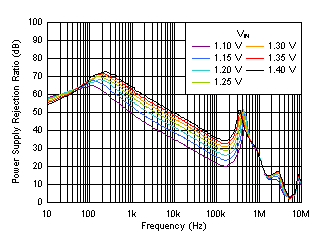IOUT = 3 A, VBIAS = 5 V, COUT = 47 µF || 10 µF || 10 µF, CNR/SS = 10 nF, CFF = 10 nF
Figure 2. PSRR vs Frequency and VIN With BiasIOUT = 1 A, COUT = 47 µF || 10 µF || 10 µF, CNR/SS = 10 nF, CFF = 10 nF
Figure 4. PSRR vs Frequency and VIN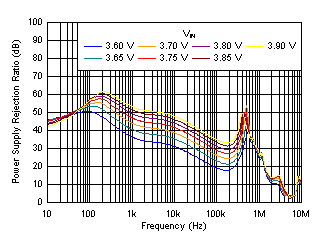IOUT = 3 A, COUT = 47 µF || 10 µF || 10 µF, CNR/SS = 10 nF, CFF = 10 nF
Figure 6. PSRR vs Frequency and VIN for VOUT = 3.3 V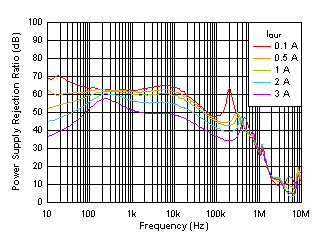VIN = VOUT + 0.6 V, COUT = 47 µF || 10 µF || 10 µF, CNR/SS = 10 nF, CFF = 10 nF
Figure 8. PSRR vs Frequency and IOUT for VOUT = 5 V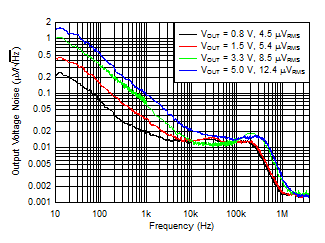VIN = VOUT + 0.4 V and VBIAS = 5 V for VOUT ≤ 2.2 V, IOUT = 4 A, COUT = 47 µF || 10 µF || 10 µF, CNR/SS = 10 nF, CFF = 10 nF, RMS noise BW = 10 Hz to 100 kHz
Figure 10. Output Voltage Noise vs Frequency and VOUTVIN = VOUT + 0.4 V, VBIAS = 5 V, IOUT = 4 A, COUT = 47 µF || 10 µF || 10 µF, CFF = 10 nF, RMS noise BW = 10 Hz to 100 kHz
Figure 12. Output Voltage Noise vs Frequency and CNR/SS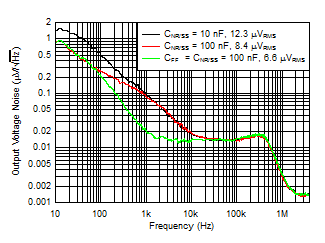VIN = 5.6 V, IOUT = 4 A, COUT = 47 µF || 10 µF || 10 µF, CFF = 10 nF, RMS noise BW = 10 Hz to 100 kHz
Figure 14. Output Voltage Noise vs Frequency at 5.0-V Output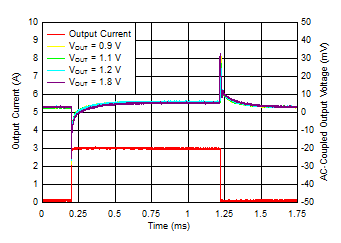VIN = VOUT + 0.3 V, VBIAS = 5 V, IOUT, DC = 100 mA, slew rate = 1 A/µs, CNR/SS = CFF = 10 nF, COUT = 47 µF || 10 µF || 10 µF
Figure 16. Load Transient vs Time and VOUT With Bias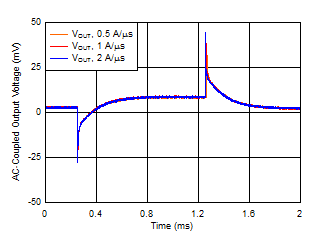VOUT = 5 V, IOUT, DC = 100 mA, IOUT = 100 mA to 4 A, COUT = 47 µF || 10 µF || 10 µF, CNR/SS = CFF = 10 nF
Figure 18. Load Transient vs Time and Slew RateIOUT = 3 A, VBIAS = 0 V
Figure 20. Dropout Voltage vs Input Voltage Without Bias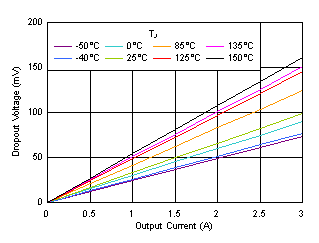VIN = 1.4 V, VBIAS = 0 V
Figure 22. Dropout Voltage vs Output Current Without Bias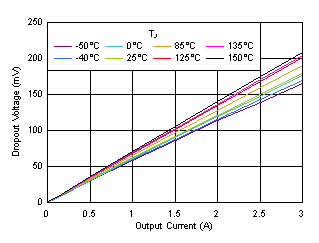VIN = 5.5 V
Figure 24. Dropout Voltage vs Output Current (High VIN)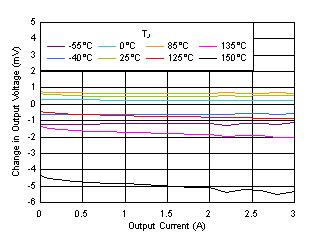VIN = 1.4 V, VBIAS = 0 V
Figure 26. Load Regulation Without Bias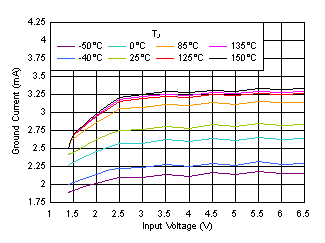VBIAS = 0 V, IOUT = 5 mA
Figure 28. Quiescent Current vs Input Voltage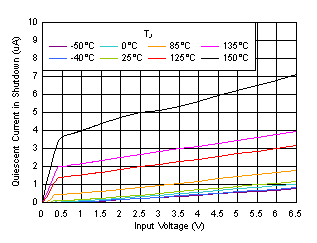VBIAS = 0 V
Figure 30. Shutdown Current vs Input Voltage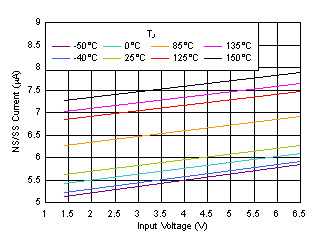VBIAS = 0 V
Figure 32. NR/SS Current vs Input Voltage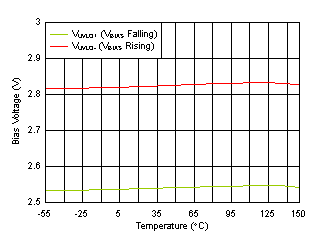VIN = 1.1 V
Figure 34. VBIAS UVLO vs TemperatureFigure 36. PG Voltage vs PG Current Sink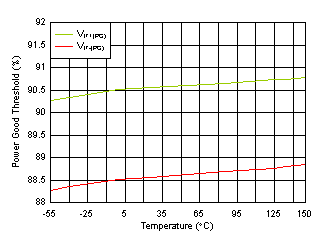Figure 38. PG Threshold vs Temperature